Next:8.3.3 Kruskal's AlgorithmUp:8.3 Minimum-Cost Spanning TreesPrevious:8.3.1 MST Property

## 8.3.2 Prim's Algorithm

This algorithm is directly based on the MST property. Assume thaV = {1, 2,..., n}.
REF.
R.C. Prim. Shortest connection networks and some generalizations. Bell System Technical Journal, Volume 36, pp. 1389-1401, 1957.

{

T =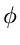;

U = { 1 };

while (U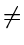V)

{

let (u, v) be the lowest cost edge

such that u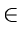U and vV - U;

T = T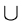{(u, v)}

U = U{v}

}

}

• See Figure 8.11 for an example.
• O(n2) algorithm.
Proof of Correctness of Prim's Algorithm

Theorem: Prim's algorithm finds a minimum spanning tree.

Proof: Let G = (V,E) be a weighted, connected graph. Let T be the edge set that is grown in Prim's algorithm. The proof is by mathematical induction on the number of edges in T and using the MST Lemma.

Basis: The empty setis promising since a connected, weighted graph always has at least one MST.

Induction Step: Assume that T is promising just before the algorithm adds a new edge e = (u,v). Let U be the set of nodes grown in Prim's algorithm. Then all three conditions in the MST Lemma are satisfied and therefore T U e is also promising.

When the algorithm stops, U includes all vertices of the graph and hence T is a spanning tree. Since T is also promising, it will be a MST.

Implementation of Prim's Algorithm

Use two arrays, closest and lowcost.

• For iV - U, closest[i] gives the vertex in U that is closest to i
• For iV - U, lowcost[i] gives the cost of the edge (i, closest(i))
•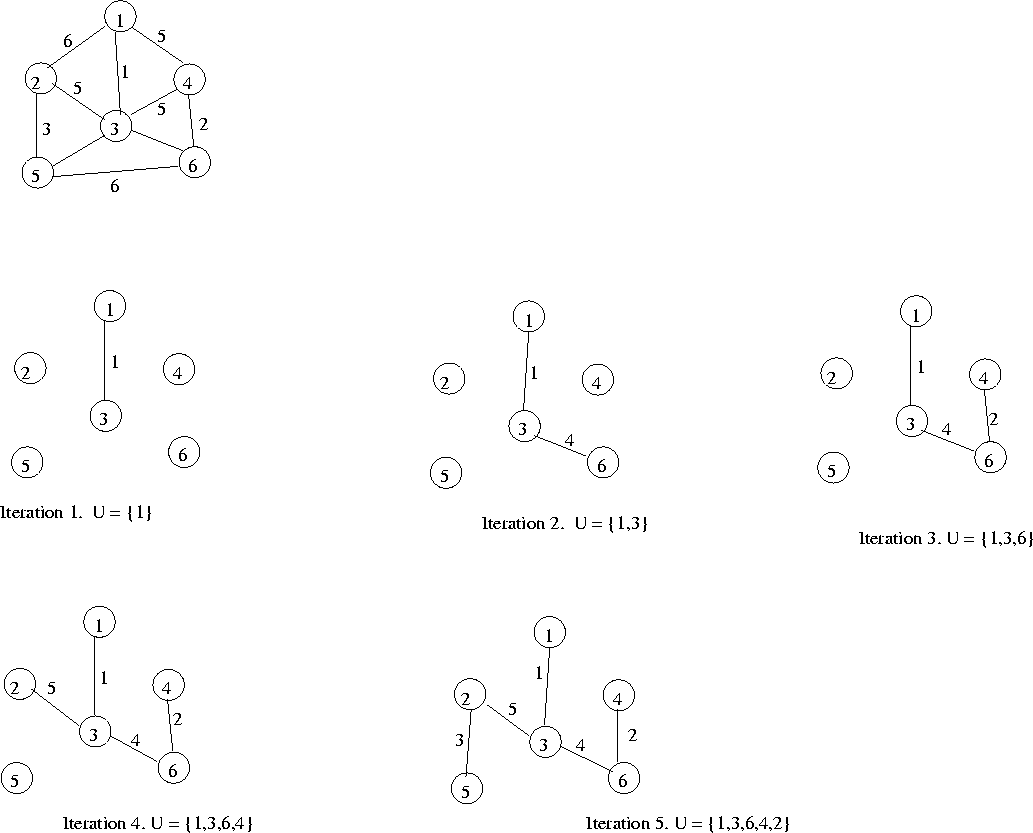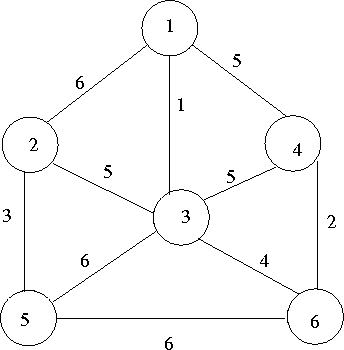• At each step, we can scan lowcost to find the vertex in V - U that is closest to U. Then we update lowcost and closest taking into account the new addition to U.
• Complexity: O(n2)
Example: Consider the digraph shown in Figure 8.12.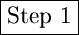U = {1} V - U = {2, 3, 4, 5, 6} closest lowcost V - U U 2 1 6 3 1 1 4 1 5 5 1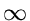6 1Select vertex 3 to include in U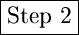U = {1, 3} V - U = {2, 4, 5, 6} closest lowcost V - U U 2 3 5 4 1 5 5 3 6 6 3 4 Now select vertex 6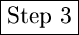U = {1, 3, 6} V - U = {2, 4, 5, 6} closest lowcost V - U U 2 3 5 4 6 2 5 3 6 Now select vertex 4, and so onNext:8.3.3 Kruskal's AlgorithmUp:8.3 Minimum-Cost Spanning TreesPrevious:8.3.1 MST Property
eEL,CSA_Dept,IISc,Bangalore